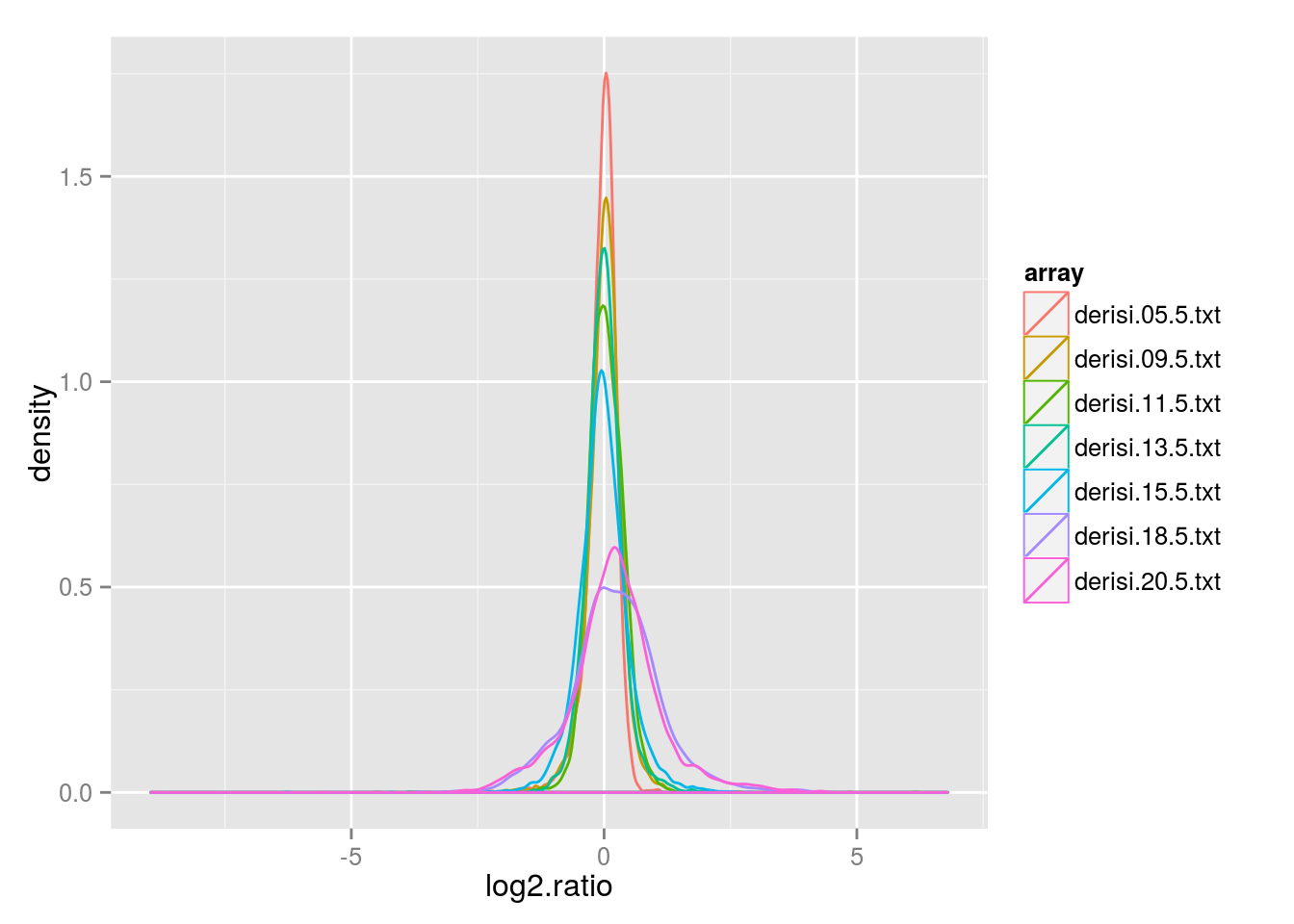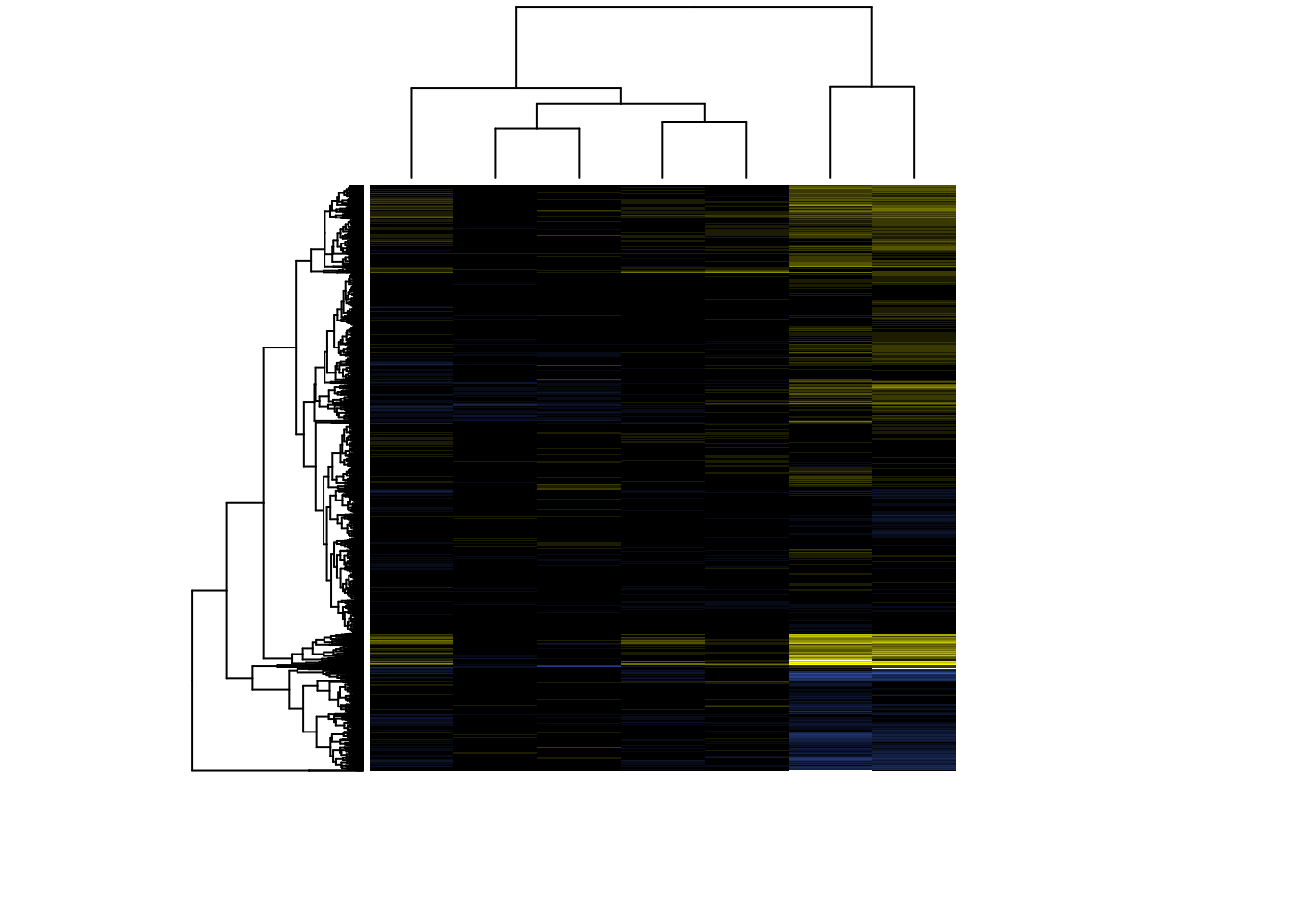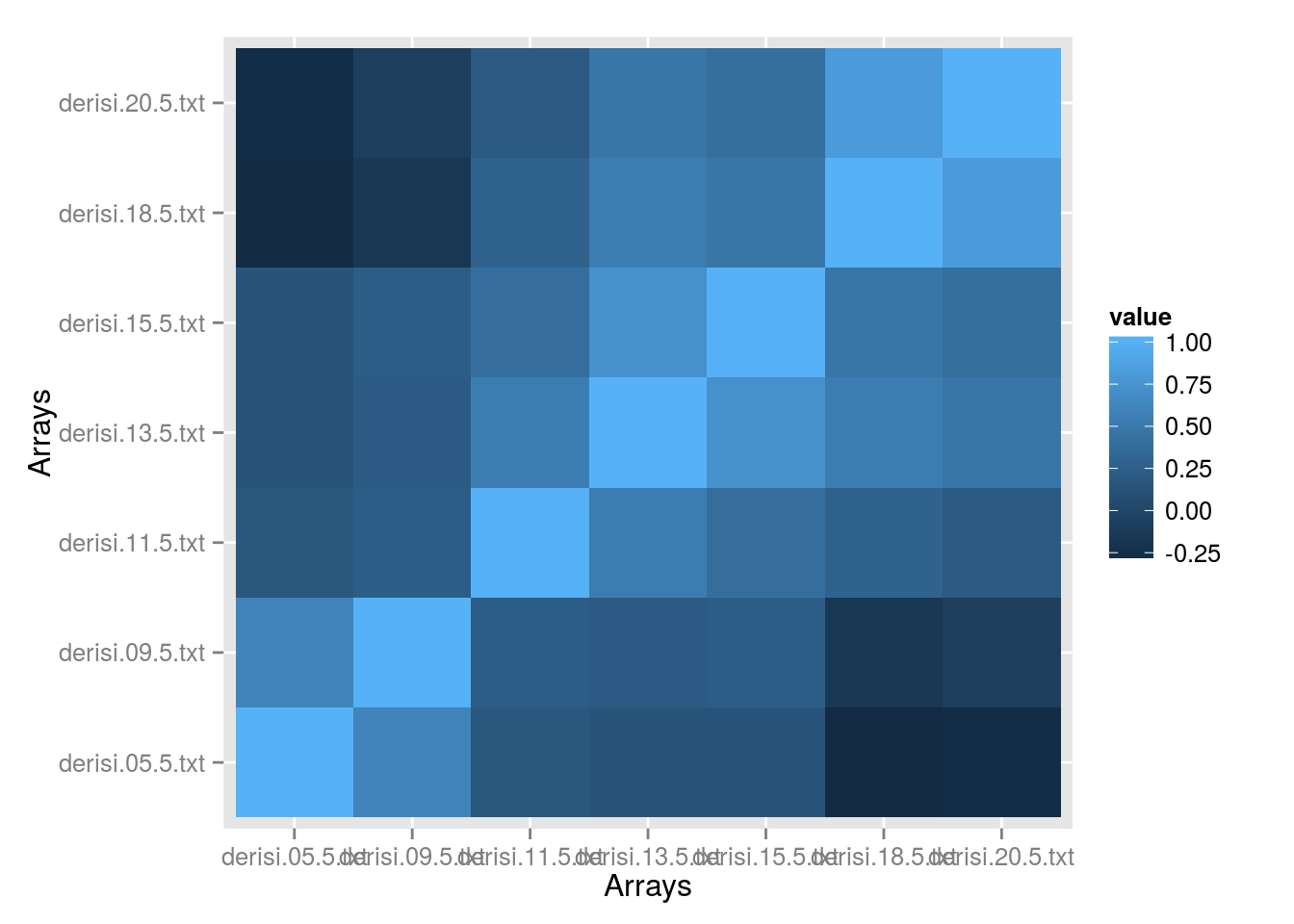The data

It would be best if you followed along with this exercise using using your project data. The only requirement is that it needs to have at least two quantitative variables. For illustration, I’m going to work with the DeRisi et al. dataset.

# Enter your code here!

For illustration, I’m going to call the data.frame of log2 transformed, normalized, expression ratios data. You should name your variable something more useful. You should check to make sure your table looks good.

##   derisi.05.5.txt derisi.09.5.txt derisi.11.5.txt derisi.13.5.txt
## 1       0.1647863       0.2714257      0.04684025      0.42867841
## 2       0.1003049       0.2363395     -0.10935876      0.14925937
## 3       0.1492594       0.4688439     -0.01449957      0.14404637
## 4      -1.1909972              NA     -0.96296927     -0.51045706
## 5      -0.7417826       0.2265085     -0.98564471     -0.04841221
## 6      -0.3510744       0.4254593     -0.57346686     -0.52076944
##   derisi.15.5.txt derisi.18.5.txt derisi.20.5.txt
## 1      0.14274017      -0.2108968       0.1826923
## 2      0.11103131      -0.1408255       0.2521734
## 3      0.07176267       0.1150332       0.2141248
## 4     -2.39592868              NA      -0.9378783
## 5     -1.27578631      -0.1942948              NA
## 6      0.40490312       0.3196179       0.2485348

Explore the raw data

As we saw in the previous microarray exercises, it’s always a good idea to start with a quick look at the distribution of raw values in the data set you’re working with. In this lab, I’ll be showing example output plots, but leave it to you to work out an implementation strategy.

We can either use the built in density and plot functons, or the ggplot2 density geom to visualize the overall distribution of value.

For example:

library(ggplot2)
## Use suppressPackageStartupMessages to eliminate package startup messages.
library(tidyr)

ggplot(gather(data, key = "array", value = "log2.ratio")) +
geom_density(aes(log2.ratio, color = array))# Enter your code here!

We can clearly see that the data have been preprocessed resulting in a distribution of expression values centered on a log2 of 0. This has implications for how we will call our hierarchical clustering function, because data (usually) need to be centered and (often) scaled before clustering.

Create a hierarchical cluster

A hierarchical cluster is an easy way to get an initial peek at the structure of a large dataset. The base function for creating a hierarchical cluster with an accompanying heatmap of the raw data matrix is heatmap.

Here are a few tricks for tweaking the clusters you make using the builtin heatmap function:

• The function expects you to pass it a matrix. You can review matrixes on the R 101 documents. You can create one with matrix or convert a data.frame to one with as.matrix.

• Use scale = "none" to suppress automatic data scaling across rows/columns. You should do this when working with this data set, since the values have already been appropriately normalized.

• You can create a custom color series for the heatmap using the colorpanel function from the gplots and pass in your custom color series using the col argument (see the scales package for a more powerful but somewhat more complicated alternative). For example, one of my favorite color series is blue-black-yellow, since it is accesible for folks with all common forms of color blindness:

library(gplots)
heats <- colorpanel( n    = 20
, low  = "royalblue3"
, mid  = "black"
, high = "yellow"
)
• Use labCol = "" and labRow="" to suppress column and row labels, respectively, when your dataset is too large for these to be readable.

• Use na.rm = FALSE to suppress removal of missing values. Play with the effects inclusion or exclusion of missing values on the overal shape of your clusters.

• Use zlim = c(-5,2) (min value, max value) to change the point at which colors are saturated (in this example any valu <= -5 is the bluest-blue and any value >=2 is the yellowest-yellow). It is ESSENTIAL to include the color color saturation values you chose when presenting a heatmap in a talk or a paper.

• Use the distfun argument to play with different ways of computing node distances (as you’ll see, the default is to use dist with it’s default of euclidean).

• Use the hclustfun argument to play with different ways of assembling the cluster nodes (the “agglomeration” method).

Make a cluster using heatmap!

Example:

m <- as.matrix(data)

#Heat map requires that there be no missing values, so we'll prune rows with NA's
m <- na.omit(m)

# Depending on the size of your data, this might take awhile
heatmap(m, col = heats, scale = "none", labRow="", labCol="",  zlim = c(-4, 4), na.rm = TRUE)# Enter your code here!

What does the structure of the cluster for the DeRisi data demonstrate about changes in gene expression over the diauxic shift time course? What does your cluter show?

Correlation matrix

A second approach to trying to find patterns in a complex data set is to calculate a correlation matrix from the raw data and then apply pattern recognition to the correlation matrix instead of the raw data. In the DeRisi case a correlation matrix could describe how similar the gene expression profiles are between the time points (column correlations) OR how similar genes across the time points (row correlations).

Fortunately, calcuating a correlation matrix is a common enough task that that aptly named cor function does it by default when passed a matrix or data.frame. Check the help file for options for the use argument.

For example:

interactionMatrix <- cor(m, use = 'pairwise.complete.obs')

Try it:

# Enter your code here!

You’ll find it’s pretty hard to visualize the distribution of values in a large matrix like this; but we already know how to solve this problem: a heatmap!

You can use the image function or use the geom_tile geom from ggplot2. ggplot expects all data to be formated in a data.frame (and we have a matrix). You can use tidyr functions to gather your matrix.

For example:

interactionTable            <- reshape2::melt(interactionMatrix)
colnames(interactionTable)  <- c( "array.1"
, "array.2"
, "value"
)

ggplot( interactionTable
, aes(array.1, array.2, fill = value)
) + geom_tile() + labs(x = "Arrays", y = "Arrays")# Enter your code here!

As expected, when the rows and columns are unordered there aren’t many obvious groups (although, you’ll notice that the order of genes in the matrix wasn’t totally random!).

Now experiment!

• Explore the efffects of using different methods to calculate the correlation matrix (the default is pearson).
• Explore the data that are invisibly returned from a call to heatmap (hint: it’s invisible, but can still be captured in a variable).
• Use cutree to perform node trimming on your cluster
• Create “zoomed in” views of subclusters with data subsetting
• Play with the effects of scaling